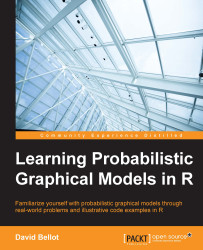•#### Learning Probabilistic Graphical Models in R#### Overview of this book

Probabilistic graphical models (PGM, also known as graphical models) are a marriage between probability theory and graph theory. Generally, PGMs use a graph-based representation. Two branches of graphical representations of distributions are commonly used, namely Bayesian networks and Markov networks. R has many packages to implement graphical models. We’ll start by showing you how to transform a classical statistical model into a modern PGM and then look at how to do exact inference in graphical models. Proceeding, we’ll introduce you to many modern R packages that will help you to perform inference on the models. We will then run a Bayesian linear regression and you’ll see the advantage of going probabilistic when you want to do prediction. Next, you’ll master using R packages and implementing its techniques. Finally, you’ll be presented with machine learning applications that have a direct impact in many fields. Here, we’ll cover clustering and the discovery of hidden information in big data, as well as two important methods, PCA and ICA, to reduce the size of big problems.
Learning Probabilistic Graphical Models in RCreditswww.PacktPub.comPrefaceFree Chapter
Probabilistic ReasoningExact InferenceLearning ParametersBayesian Modeling – Basic ModelsApproximate InferenceBayesian Modeling – Linear ModelsProbabilistic Mixture ModelsAppendixIndex## Chapter 5. Approximate Inference

This chapter introduces a second class of inference algorithms, maybe the most important of all because of their versatility. The approach is completely different from what we have seen until now. Indeed, we saw two classes of algorithms, one based on a pure analytic resolution of the problem by calculating manually the posterior distribution and the other one by using message propagation in a graph. In both cases, the result was computed exactly. In the case of an analytic solution, computing the solution usually boils down to computing a function of the posterior distribution. In the case of a message-passing algorithm, computing the posterior distribution is done step-by-step by propagating messages on a graph. If the graph is not appropriate for this type of algorithm, the computations can be extremely long and often intractable.

However, in many cases, we can trade a bit of accuracy for more speed. This is the main idea of approximate inference. Does...